# Dimensionality Reduction

#### 4/10/2020

# load python
library(reticulate)
use_condaenv("my_ml")
# load packages
import numpy as np
from sklearn.datasets import fetch_openml
from sklearn.model_selection import train_test_split
from sklearn.preprocessing import StandardScaler
from sklearn.decomposition import PCA
import pandas as pd
import matplotlib.pyplot as plt
import seaborn as sns; sns.set()
import time
import xgboost as xgb
from sklearn import metrics
from sklearn.metrics import confusion_matrix, classification_report, f1_score

# 1 Introduction to Dimensionality Reduction

Many times, the type of data that we have and use to answer most types of quantitative research questions is of large $$N$$ and small $$k$$. Other types of research questions, such as Genome classification or natural language processing, involve high-dimensional data. In these situations, $$k$$ can approach $$N$$ and therefore lead to overfitting situations due to near perfect correlations.

The idea behind dimensionality reduction is simple: take high dimensional feature spaces ($$k$$) and project them onto lower dimensional subspaces ($$m$$) (where $$m$$ < $$k$$). Dimensionality reduction has several kind of appealing properties like solving the curse of dimensionality and overfitting, but it also allows us to visualize high dimensional data and to compress it. Collapsing high dimensional data that would otherwise be too difficult for us to understand or interpret suddenly becomes much more salient when we collapse it down into two or three dimensions.

The goal behind dimensionality reduction algorithms is to minimize the loss of information. We want to find new dimensions that still accurately represent the variation in the original data. Consider the plot below, which shows some simulated data that we can imagine is height (y) and weight (x) for women. While we have height and weight, what we really want is just size, thereby transforming two dimensions into one.

# simulate data
x <- rnorm(100, 120, 5)  # obs, mean, std
u <- rnorm(100, 5, 1)
y <- 1 + 0.5 * x + u
fit1 <- lm(y ~ x)
plot(x, y)
abline(fit1)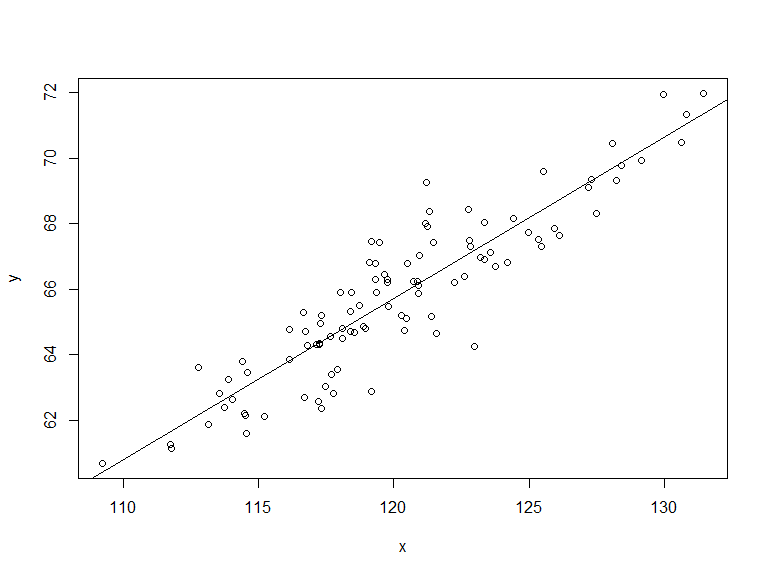Our goal, then, is to find the line with the least loss of information which means that we need to find a line that captures as much variance as possible. The line that captures as much variance of this data is something that looks a little bit like the regression line (but it is not the regression line).

When you project the original data points onto the line that captures the most variance, the projection will spread out the original data points across this new axis $$z$$ to the maximum extent possible.

In this applied demonstration, we will focus on a method called PCA, or principal component analysis, which is the most common form of dimensionality reduction. For statisticians and econometricians, PCA is probably the canonical form of unsupervised learning along with cluster analysis. PCA is often a pre-processing step used by data scientists.

# 2 Principal Component Analysis: Overview

The idea behind PCA is to find a lower-dimensional surface onto which you can project your original data in a way that minimizes this projection error. Projection error is the sum of squared distances between the between the observations and the line that maximizes the data’s variance.

The distance is the orthogonal distance between the observation and the line. This is what makes it different from linear regression, where the sum of squared errors is the vertical distance between the point and the line.

This example shows a three-dimensional space with points that are red and blue and green. Next to it is an image of a two-dimensional hyperplane that we use to take the projections of these points onto two-dimensional space. By looking at the hyperplane, we can see how principal components reveal slightly different structure to the data than originally seen.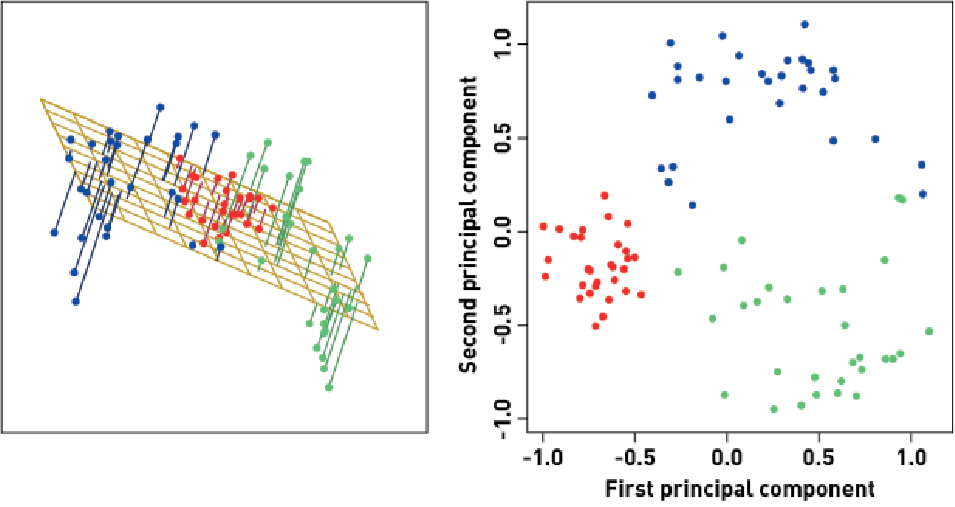Example

# 3 Principal Component Analysis: How It Works

The goal is to find the vectors, or the principal components, and project them onto a lower dimensional subspace. The principal components of the original data set is determined by the first $$m$$ eigenvector of the covariance matrix of the data. Put differently, the principal components of your high dimensional data set in k dimensions are the first m, where m is less than k, eigenvectors of the covariance matrix of that data set. Eigenvectors and eigenvalues, a special set of vectors that characterize a matrix of data, are a comparable way of summarizing data in a matrix.

The idea behind principal component analysis is to diagonalize the covariance matrix of the normalized original data. This returns the eigenvectors, which are the directions of the axes of your new projections, and the eigenvalues tell you the amount of variance captured by each of those eigenvectors.

## 3.1 Step 1:

Compute the covariance matrix of the data.

## 3.2 Step 2:

Compute the eigenvectors via the singular value decomposition algorithm, returning three matrices: U, $$\Sigma$$, and V.

• U: An $$n$$ by $$m$$ unitary matrix, where $$m$$ is the dimensionality of the space that we are projecting onto. The columns of U are the eigenvectors, representing the direction of the greatest variation in the data.

• $$\Sigma$$: An $$n$$ by $$n$$ diagonal matrix of singular values where $$n$$ is the number of observations. The non-zero diagonal elements of $$\Sigma$$ are the square roots of the non-zero eigenvalues.

• V: An $$n$$ by $$n$$ unitary matrix that contains vectors defining each principal component.

We can derive them manually like so:

# load sklearn digits data
print(digits.data.shape)

X = digits["data"]

X_centered = X - X.mean(axis=0)  # required if done manually
U, s, V = np.linalg.svd(X_centered)
c1 = V.T[:, 0]  # vector for first independent var
c2 = V.T[:, 1]  # vector for second independent var

## 3.3 Step 3: Project onto hyperplane

Lastly, we can project our first 10 principal components onto a hyperplane like so:

projected_X = V.T[:, :10]
projected_X_reduced = X_centered.dot(projected_X)

# 4 PCA in Practice

Before starting we want to normalize our data which typically means that we subtract the mean and divide by the standard deviation (if we use sklearn, it will do this for us). Next, we want to determine what our goal is in dimensionality reduction. If we seek to visualize high-dimensional data, then we want to project our data down to two or maybe three dimensions. If we seek to perform some unsupervised learning, there are often natural breaking points in the data that can help you determine what the optimal space is to project onto, which is a little bit different from k means clustering where you can rely on these metrics like intercluster correlation and separation between clusters.

## 4.1 PCA: Visualization

The utility of reducing high dimensional data onto lower dimensions is demonstrated below by drawing on sklearn’s digits data. Notice that the data is comprised of 8x8 pixels which means that they are 64 dimensional.

# load sklearn digits data
print(digits.data.shape)
## (1797, 64)
X = digits["data"]
y = digits["target"]
X_train, X_test, y_train, y_test = train_test_split(X, y)

Next we can instantiate PCA like so:

pca = PCA(2)  # project from 64 to 2 dimensions
projected = pca.fit_transform(digits.data)
print(digits.data.shape)
## (1797, 64)
print(projected.shape)
## (1797, 2)

And plot our two dimensional projected data:

plt.scatter(projected[:, 0], projected[:, 1],
c=digits.target, edgecolor='none', alpha=0.5,
cmap=plt.cm.get_cmap('Spectral', 10))
plt.xlabel('component 1')
plt.ylabel('component 2')
plt.colorbar();

We have found the optimal stretch and rotation in 64-dimensional space that allows us to see the layout of the digits in two dimensions, and we have done this in an unsupervised manner.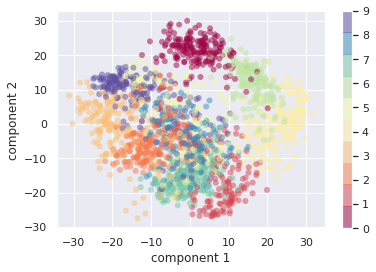64 to 2 Dimensions

## 4.2 PCA: Retained Variance

With PCA, its easier to figure out how many components we want by using the retained variance statistic which is the average squared projection error divided by the total variation in the data. In practice, we select some percentage of the retained variance that we want to capture and run PCA to then choose the number of components that exceed our threshold percentage.

To find retained variance, we compute the following:

pca = PCA().fit(digits.data)
plt.plot(np.cumsum(pca.explained_variance_ratio_))
plt.xlabel('number of components')
plt.ylabel('cumulative explained variance');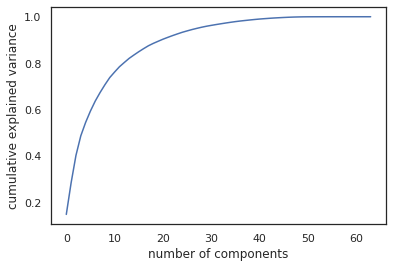Retained Variance

This curve quantifies how much of the total, 64-dimensional variance is contained within the first $$N$$ components. For example, we see that with the digits the first 10 components contain approximately 75% of the variance, while you need around 50 components to describe close to 100% of the variance.

So, if we want to preserve 50% of the total variance, we can run:

pca = PCA(n_components=0.5)  # preserve 50% of variance
X_reduced = pca.fit_transform(X_train)  # fit on training data

Further, if we want to re-transform our data back to its original dimensions, we can, so long as we are comfortable with losing some amount of variation and thus data quality; as evidenced by the pictures below.

pca = PCA(n_components=0.5)
X_reduced = pca.fit_transform(X_train)
X_recovered = pca.inverse_transform(X_reduced)

print(X_reduced.shape)
print(X_recovered.shape)

def plot_digits(data):
# 4 rows, 10 cols
fig, axes = plt.subplots(4, 10, figsize=(10, 4),
subplot_kw={'xticks':[], 'yticks':[]},
gridspec_kw=dict(hspace=0.1, wspace=0.1))
for i, ax in enumerate(axes.flat):
ax.imshow(data[i].reshape(8, 8),
cmap='binary', interpolation='nearest',
clim=(0, 16))
plot_digits(X_train)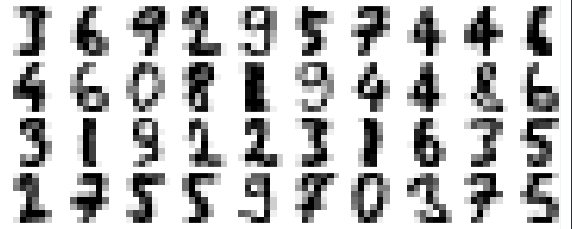Original

plot_digits(X_recovered)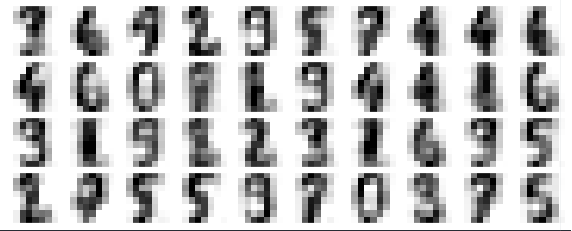Recovered

# 5 PCA Classification: MNIST

In this section, we apply PCA to the MNIST data set to provide a short walk through of PCA used in practice. In the chunk below, we begin by loading our data using sklearn’s handy package to access MNIST. We then shuffle, split, and scale our data.

# load MNIST from https://www.openml.org/d/554 via fetch_openml
X, y = fetch_openml(name='mnist_784', return_X_y=True, cache=False)

# shuffle the data
shuffle = np.random.permutation(np.arange(X.shape))  # generate random indices
X, y = X[shuffle], y[shuffle]  # apply shuffle

X = X.astype('float32')  # transform float32
y = y.astype('float32')  # transform float32

# split data
X_train_full, X_test, y_train_full, y_test = train_test_split(X, y, random_state=42)
X_train, X_dev, y_train, y_dev = train_test_split(X_train_full, y_train_full, random_state=42)

# standardize
scaler = StandardScaler()
X_train_full = scaler.fit_transform(X_train_full)
X_train = scaler.transform(X_train)
X_dev = scaler.transform(X_dev)
X_test = scaler.transform(X_test)

Notice that we have 784 dimensional data, owing to 64x64 pixel images.

print(X.shape)
## (70000, 784)

Our first step is to examine our data by looking at the ratio of components to explained variance. We can see that roughly 150 components provide us with over 90% of our data’s variation.

# check total variance by component
pca = PCA().fit(X)
plt.plot(np.cumsum(pca.explained_variance_ratio_))
plt.xlabel('number of components')
plt.ylabel('cumulative explained variance')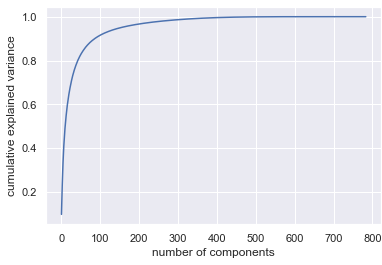MNIST: Explained Variance:Components

Since we are dealing with high dimensional data, we can take a look at how our data looks like in two dimensions by the following code:

# visualize MNIST in two dimensions
pca = PCA(2)  # project from 784 to 2 dimensions
projected = pca.fit_transform(X)

plt.scatter(projected[:, 0], projected[:, 1],
c=y, edgecolor='none', alpha=0.5,
cmap=plt.cm.get_cmap('Spectral', 10))
plt.xlabel('component 1')
plt.ylabel('component 2')
plt.colorbar();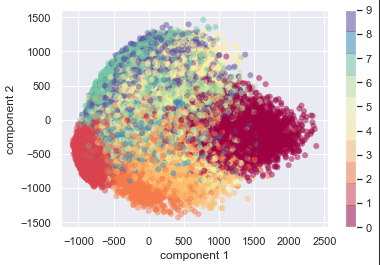MNIST: In Two Dimensions

Next, we preprocess our data for analysis by having PCA retain just the number of dimensions that yield us roughly 90% of the total variation.

# transform training data
pca = PCA(n_components=0.9)  # preserve 90% of variance
X_train_reduced = pca.fit_transform(X_train)  # fit on training data
X_dev_reduced = pca.transform(X_dev) # transform dev data
X_test_reduced = pca.transform(X_test) # transform test data
# create xgboost decision tree
xgb_tree = xgb.XGBClassifier(objective='multi:softmax', learning_rate=1,
max_depth=2, alpha=1, n_estimators=100,
booster='gbtree')
# fit the model
start = time.time()
xgb_tree.fit(X_train_reduced, y_train,
eval_set=[(X_dev_reduced, y_dev)],
eval_metric='merror',
early_stopping_rounds = 7)
##   validation_0-merror:0.40427
## Will train until validation_0-merror hasn't improved in 7 rounds.
##   validation_0-merror:0.31802
##   validation_0-merror:0.26918
##   validation_0-merror:0.23520
##   validation_0-merror:0.20823
##   validation_0-merror:0.19116
##   validation_0-merror:0.17707
##   validation_0-merror:0.16571
##   validation_0-merror:0.15871
##   validation_0-merror:0.15063
##  validation_0-merror:0.14423
##  validation_0-merror:0.13669
##  validation_0-merror:0.13333
##  validation_0-merror:0.12968
##  validation_0-merror:0.12518
##  validation_0-merror:0.12015
##  validation_0-merror:0.11840
##  validation_0-merror:0.11497
##  validation_0-merror:0.11055
##  validation_0-merror:0.10918
##  validation_0-merror:0.10705
##  validation_0-merror:0.10453
##  validation_0-merror:0.10217
##  validation_0-merror:0.09981
##  validation_0-merror:0.09867
##  validation_0-merror:0.09676
##  validation_0-merror:0.09570
##  validation_0-merror:0.09288
##  validation_0-merror:0.09166
##  validation_0-merror:0.08937
##  validation_0-merror:0.08823
##  validation_0-merror:0.08815
##  validation_0-merror:0.08785
##  validation_0-merror:0.08739
##  validation_0-merror:0.08671
##  validation_0-merror:0.08518
##  validation_0-merror:0.08427
##  validation_0-merror:0.08495
##  validation_0-merror:0.08389
##  validation_0-merror:0.08267
##  validation_0-merror:0.08107
##  validation_0-merror:0.08015
##  validation_0-merror:0.08008
##  validation_0-merror:0.07992
##  validation_0-merror:0.07870
##  validation_0-merror:0.07749
##  validation_0-merror:0.07680
##  validation_0-merror:0.07703
##  validation_0-merror:0.07741
##  validation_0-merror:0.07589
##  validation_0-merror:0.07581
##  validation_0-merror:0.07459
##  validation_0-merror:0.07459
##  validation_0-merror:0.07459
##  validation_0-merror:0.07390
##  validation_0-merror:0.07383
##  validation_0-merror:0.07451
##  validation_0-merror:0.07368
##  validation_0-merror:0.07261
##  validation_0-merror:0.07246
##  validation_0-merror:0.07208
##  validation_0-merror:0.07162
##  validation_0-merror:0.07139
##  validation_0-merror:0.07139
##  validation_0-merror:0.07131
##  validation_0-merror:0.07048
##  validation_0-merror:0.07086
##  validation_0-merror:0.06994
##  validation_0-merror:0.06971
##  validation_0-merror:0.06827
##  validation_0-merror:0.06888
##  validation_0-merror:0.06895
##  validation_0-merror:0.06811
##  validation_0-merror:0.06872
##  validation_0-merror:0.06857
##  validation_0-merror:0.06773
##  validation_0-merror:0.06781
##  validation_0-merror:0.06743
##  validation_0-merror:0.06705
##  validation_0-merror:0.06591
##  validation_0-merror:0.06667
##  validation_0-merror:0.06613
##  validation_0-merror:0.06636
##  validation_0-merror:0.06613
##  validation_0-merror:0.06545
##  validation_0-merror:0.06537
##  validation_0-merror:0.06469
##  validation_0-merror:0.06469
##  validation_0-merror:0.06423
##  validation_0-merror:0.06423
##  validation_0-merror:0.06438
##  validation_0-merror:0.06400
##  validation_0-merror:0.06331
##  validation_0-merror:0.06316
##  validation_0-merror:0.06232
##  validation_0-merror:0.06263
##  validation_0-merror:0.06171
##  validation_0-merror:0.06156
##  validation_0-merror:0.06164
##  validation_0-merror:0.06164
## XGBClassifier(alpha=1, base_score=0.5, booster='gbtree', colsample_bylevel=1,
##               colsample_bynode=1, colsample_bytree=1, gamma=0, gpu_id=-1,
##               importance_type='gain', interaction_constraints=None,
##               learning_rate=1, max_delta_step=0, max_depth=2,
##               min_child_weight=1, missing=nan, monotone_constraints=None,
##               n_estimators=100, n_jobs=0, num_parallel_tree=1,
##               objective='multi:softprob', random_state=0, reg_alpha=1,
##               reg_lambda=1, scale_pos_weight=None, subsample=1,
##               tree_method=None, validate_parameters=False, verbosity=None)
end = time.time()
print('Elapsed Training Time:', end - start)
## Elapsed Training Time: 582.6203162670135
predicted_y = xgb_tree.predict(X_test_reduced, ntree_limit=xgb_tree.best_iteration)
print(classification_report(y_test, predicted_y))
##               precision    recall  f1-score   support
##
##          0.0       0.97      0.96      0.97      1696
##          1.0       0.98      0.97      0.98      1926
##          2.0       0.92      0.94      0.93      1714
##          3.0       0.92      0.91      0.91      1815
##          4.0       0.93      0.93      0.93      1722
##          5.0       0.91      0.91      0.91      1595
##          6.0       0.96      0.97      0.96      1737
##          7.0       0.95      0.93      0.94      1838
##          8.0       0.91      0.90      0.90      1718
##          9.0       0.91      0.93      0.92      1739
##
##     accuracy                           0.94     17500
##    macro avg       0.94      0.94      0.94     17500
## weighted avg       0.94      0.94      0.94     17500
print(confusion_matrix(y_test, predicted_y))
## [[1630    0   11    7    5    8   16    4   14    1]
##  [   0 1876   18    3    4    3    4    4   13    1]
##  [  10    2 1613   25   10    7    5   21   19    2]
##  [   2    4   42 1645    3   60    0   13   31   15]
##  [   6    8    9    2 1607    3   14    9   11   53]
##  [  10    6    9   33    8 1453   20   11   34   11]
##  [   9    0    9    3    8   17 1683    0    8    0]
##  [   3    5   24   10   20    5    0 1710    6   55]
##  [   4   14   11   48   13   36   10    9 1549   24]
##  [   4    2    6   15   42    8    1   26   26 1609]]

## 5.1 Evaluating Training Time Differences

In this section, we run the model again to show the difference in training time and predictive results has on our choice to use dimensionality reduction.

# fit the model
start = time.time()
xgb_tree.fit(X_train, y_train,
eval_set=[(X_dev, y_dev)],
eval_metric='merror',
early_stopping_rounds = 7)
##   validation_0-merror:0.41844
## Will train until validation_0-merror hasn't improved in 7 rounds.
##   validation_0-merror:0.29699
##   validation_0-merror:0.24998
##   validation_0-merror:0.21181
##   validation_0-merror:0.19558
##   validation_0-merror:0.17409
##   validation_0-merror:0.15855
##   validation_0-merror:0.14811
##   validation_0-merror:0.13966
##   validation_0-merror:0.12937
##  validation_0-merror:0.12556
##  validation_0-merror:0.12023
##  validation_0-merror:0.11444
##  validation_0-merror:0.11078
##  validation_0-merror:0.10598
##  validation_0-merror:0.10293
##  validation_0-merror:0.10111
##  validation_0-merror:0.09730
##  validation_0-merror:0.09341
##  validation_0-merror:0.09021
##  validation_0-merror:0.08815
##  validation_0-merror:0.08571
##  validation_0-merror:0.08480
##  validation_0-merror:0.08213
##  validation_0-merror:0.08137
##  validation_0-merror:0.07817
##  validation_0-merror:0.07665
##  validation_0-merror:0.07528
##  validation_0-merror:0.07429
##  validation_0-merror:0.07299
##  validation_0-merror:0.07169
##  validation_0-merror:0.07116
##  validation_0-merror:0.06926
##  validation_0-merror:0.06850
##  validation_0-merror:0.06750
##  validation_0-merror:0.06811
##  validation_0-merror:0.06773
##  validation_0-merror:0.06720
##  validation_0-merror:0.06537
##  validation_0-merror:0.06484
##  validation_0-merror:0.06461
##  validation_0-merror:0.06278
##  validation_0-merror:0.06270
##  validation_0-merror:0.06149
##  validation_0-merror:0.06194
##  validation_0-merror:0.06103
##  validation_0-merror:0.05981
##  validation_0-merror:0.05973
##  validation_0-merror:0.05912
##  validation_0-merror:0.05905
##  validation_0-merror:0.05821
##  validation_0-merror:0.05729
##  validation_0-merror:0.05684
##  validation_0-merror:0.05638
##  validation_0-merror:0.05623
##  validation_0-merror:0.05585
##  validation_0-merror:0.05486
##  validation_0-merror:0.05470
##  validation_0-merror:0.05379
##  validation_0-merror:0.05356
##  validation_0-merror:0.05326
##  validation_0-merror:0.05349
##  validation_0-merror:0.05310
##  validation_0-merror:0.05333
##  validation_0-merror:0.05249
##  validation_0-merror:0.05257
##  validation_0-merror:0.05219
##  validation_0-merror:0.05189
##  validation_0-merror:0.05067
##  validation_0-merror:0.05021
##  validation_0-merror:0.04990
##  validation_0-merror:0.04914
##  validation_0-merror:0.04960
##  validation_0-merror:0.04846
##  validation_0-merror:0.04838
##  validation_0-merror:0.04846
##  validation_0-merror:0.04815
##  validation_0-merror:0.04747
##  validation_0-merror:0.04709
##  validation_0-merror:0.04709
##  validation_0-merror:0.04709
##  validation_0-merror:0.04739
##  validation_0-merror:0.04701
##  validation_0-merror:0.04587
##  validation_0-merror:0.04609
##  validation_0-merror:0.04617
##  validation_0-merror:0.04579
##  validation_0-merror:0.04518
##  validation_0-merror:0.04510
##  validation_0-merror:0.04503
##  validation_0-merror:0.04480
##  validation_0-merror:0.04495
##  validation_0-merror:0.04495
##  validation_0-merror:0.04503
##  validation_0-merror:0.04427
##  validation_0-merror:0.04427
##  validation_0-merror:0.04373
##  validation_0-merror:0.04389
##  validation_0-merror:0.04358
##  validation_0-merror:0.04320
## XGBClassifier(alpha=1, base_score=0.5, booster='gbtree', colsample_bylevel=1,
##               colsample_bynode=1, colsample_bytree=1, gamma=0, gpu_id=-1,
##               importance_type='gain', interaction_constraints=None,
##               learning_rate=1, max_delta_step=0, max_depth=2,
##               min_child_weight=1, missing=nan, monotone_constraints=None,
##               n_estimators=100, n_jobs=0, num_parallel_tree=1,
##               objective='multi:softprob', random_state=0, reg_alpha=1,
##               reg_lambda=1, scale_pos_weight=None, subsample=1,
##               tree_method=None, validate_parameters=False, verbosity=None)
end = time.time()
print('Elapsed Training Time:', end - start)
## Elapsed Training Time: 832.9003236293793
predicted_y = xgb_tree.predict(X_test, ntree_limit=xgb_tree.best_iteration)
print(classification_report(y_test, predicted_y))
##               precision    recall  f1-score   support
##
##          0.0       0.97      0.97      0.97      1696
##          1.0       0.97      0.98      0.98      1926
##          2.0       0.94      0.96      0.95      1714
##          3.0       0.95      0.93      0.94      1815
##          4.0       0.95      0.95      0.95      1722
##          5.0       0.95      0.94      0.94      1595
##          6.0       0.96      0.97      0.96      1737
##          7.0       0.97      0.95      0.96      1838
##          8.0       0.94      0.94      0.94      1718
##          9.0       0.93      0.94      0.94      1739
##
##     accuracy                           0.95     17500
##    macro avg       0.95      0.95      0.95     17500
## weighted avg       0.95      0.95      0.95     17500
print(confusion_matrix(y_test, predicted_y))
## [[1643    1   10    2    7    3   12    0   16    2]
##  [   0 1890   12    3    5    1    4    4    5    2]
##  [   4    8 1642   15    7    3    5   13   14    3]
##  [   3    8   30 1697    4   30    0   13   20   10]
##  [   2    7    8    3 1629    3   15    6    5   44]
##  [   8    7    5   29    4 1494   22    1   17    8]
##  [  13    0    4    1    5   21 1684    1    7    1]
##  [   5    9   20   11   14    1    0 1745    7   26]
##  [   5   10   13   21   10   19   11    2 1607   20]
##  [   7    4    5    6   28    3    1   23   20 1642]]

# 6 Sources

• Matthias Feurer, Jan N. van Rijn, Arlind Kadra, Pieter Gijsbers, Neeratyoy Mallik, Sahithya Ravi, Andreas Mueller, Joaquin Vanschoren, Frank Hutter. OpenML-Python: an extensible Python API for OpenML. arXiv:1911.02490 [cs.LG], 2019

• LeCun, Yann. “The MNIST database of handwritten digits.” http://yann.lecun.com/exdb/mnist/ (1998).